Get instant live expert help with Excel or Google Sheets“My Excelchat expert helped me in less than 20 minutes, saving me what would have been 5 hours of work!”

#### Post your problem and you’ll get Expert help in seconds.

Your message must be at least 40 characters
Our professional Expert are available now. Your privacy is guaranteed.

# First Match Between Two Ranges

Excel allows us to find the first match between two ranges with INDEX, MATCH and COUNTIF functions. This step by step tutorial will assist all levels of Excel users to learn how to get the first matchable value from the two arrays.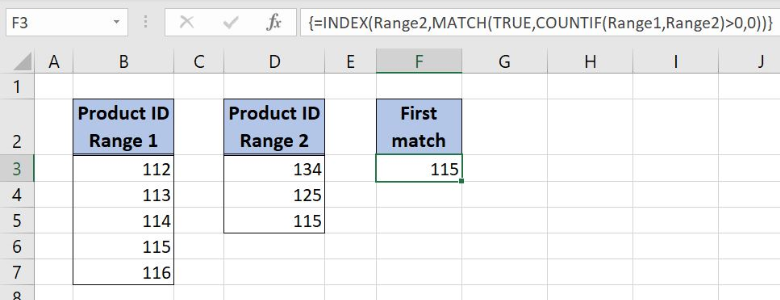Figure 1. Get the first match between two ranges

## Syntax of the INDEX Formula

`=INDEX(array, row_num, column_num)`

The parameters of the INDEX function are:

• array – a range of cells where we want to get a data
• row_num – a number of a row in the array for which we want to get a value
• column_num – a column in the array which returns a value.

## Syntax of the MATCH Formula

`=MATCH(lookup_value, lookup_array, [match_type])`

The parameters of the MATCH function are:

• lookup_value – a value which we want to find in the lookup_array
• lookup_array – the array where we want to find a value
• [match_type] – a type of match. We put 0 which is an exact match.

## Syntax of the COUNTIF Formula

The generic formula for the COUNTIF function is:

` =COUNTIF(range,criteria)`

The parameters of the COUNTIF function are:

• range – ranges where we want to apply our criteria
• criteria – a criteria in the range which we want to count.

## Setting up Our Data to Find the First Match of Two Ranges

Our first table consists of 1 column “Product ID Range 1” (column B) while the second one has 1 column “Product ID Range 2” (column D). The idea is to find the first matchable number from Column B with the numbers from the Column D and to place the result in the cell F3.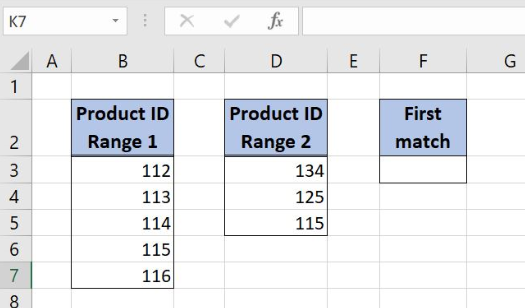Figure 2. Data that we will use in the example

## Get the First Match Between Two Ranges with INDEX, MATCH, and COUNTIF Functions

We want to get the first match between the two tables and to place the result in the cell F3. In order to make the formula more clear, we will create a named range Range1 for cell range B3:B7 and Range2 for cell range D3:D5.

To create a named range we should follow the steps:

• Select the cell range that should be named
• Click on the name box in Excel
• Write the name for the cell range and press enter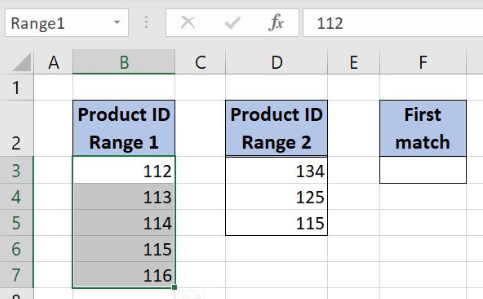Figure 3. Creating a named range Range1 for column “Product ID Range 1”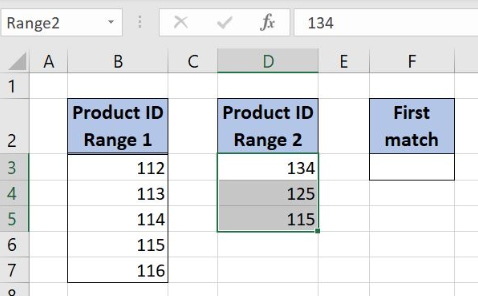Figure 4. Creating a named range Range2 for column “Product ID Range 2”

The formula looks like:

`{=INDEX(Range2,MATCH(TRUE,COUNTIF(Range1,Range2)>0,0))}`

The array parameter of the INDEX function is a named range Range2 while the row_num is the formula part `MATCH(TRUE,COUNTIF(Range1,Range2)>0,0)`.

In MATCH function the  lookup_value is TRUE while the lookup_array is the formula part `COUNTIF(Range1,Range2)>0`. The match_type is 0 since we want the exact match. Finally, the range parameter of the COUNTIF function is the named range Range1, while the criteria is the named range Range2.

To apply the formula, we need to follow these steps:

• Select cell F3 and click on it
• Insert the formula: `=INDEX(Range2,MATCH(TRUE,COUNTIF(Range1,Range2)>0,0))`,
• Press ctrl+shift+enter to convert the formula to the array function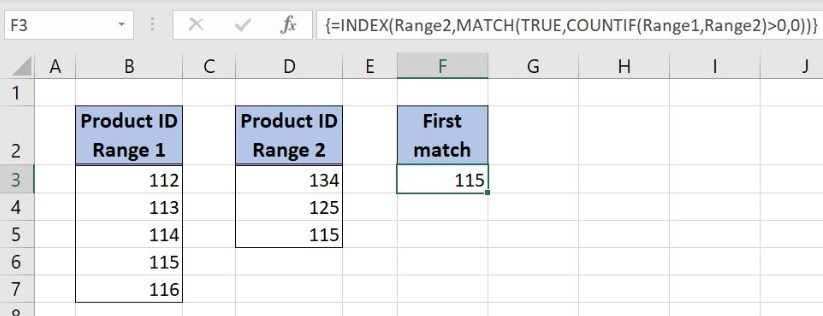Figure 5. Get the first match using the INDEX, MATCH and COUNTIF functions

With INDEX function we want to get the first matchable number from the Range2 with the numbers from the Range1. MATCH function result is giving us the information about the row_num parameter of the INDEX function.

In MATCH function lookup_value is TRUE while the lookup_array is the result of the COUNTIF function. COUNTIF function is checking if each value from the Range2 exists in the Range1. The result is 1 if the number from the Range2 exists in the Range1 and value 0 if not: `{0,0,1}`.

Formula part {0;0;1}>0 is checking if the each value in the COUNTIF output array is greater than 0: `{FALSE,FALSE,TRUE}`. This array is the lookup_array for the MATCH function: `MATCH(TRUE,{FALSE,FALSE,TRUE},0)`. MATCH function result is number 3, and the total formula result is the value 115 from the Range2 because it is the third value in the range.

Most of the time, the problem you will need to solve will be more complex than a simple application of a formula or function. If you want to save hours of research and frustration, try our live Excelchat service! Our Excel Experts are available 24/7 to answer any Excel question you may have. We guarantee a connection within 30 seconds and a customized solution within 20 minutes.

### Did this post not answer your question? Get a solution from connecting with the expert.Another blog reader asked this question today on Excelchat:
Solution examplesI need a formula to compare the data in two columns and then export the mismatched data in the 3rd column
Solved by S. Q. in 20 minsUse the Vlookup Function to complete the "employee" column of table 2. Use "job Id" from table 2 as your lookup_value(s) and table 1 as your reference.
Solved by C. H. in 16 minsIf a cell in another sheet is populated I need a vlookup done. If the cell is not populated I need the cell to return blank.
Solved by T. D. in 60 minsI am trying to make a chart that turns a week range red if nothing is entered in the range. If something is entered then I would like it to turn green. Please Help
Solved by E. U. in 43 minsI need a check box to show/hide an answer of an if function
Solved by Z. U. in 23 mins## Subscribe to Excelchat.coAnother blog reader asked this question today on Excelchat: